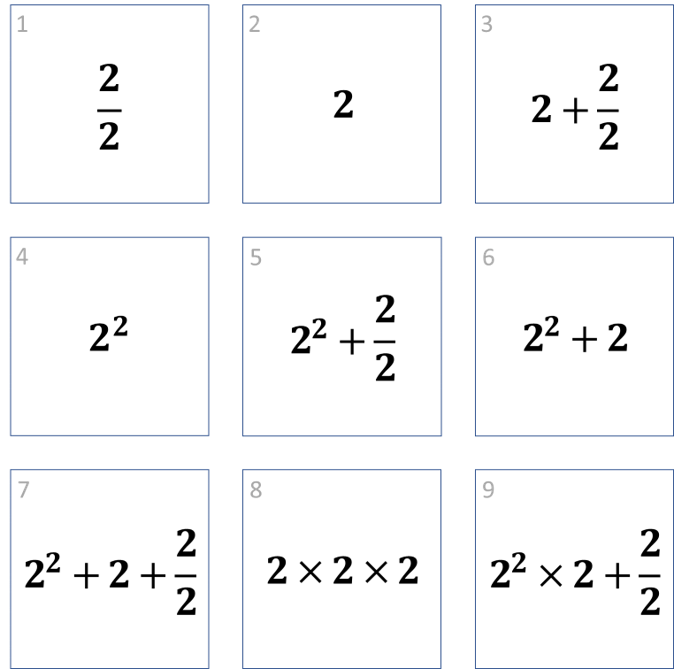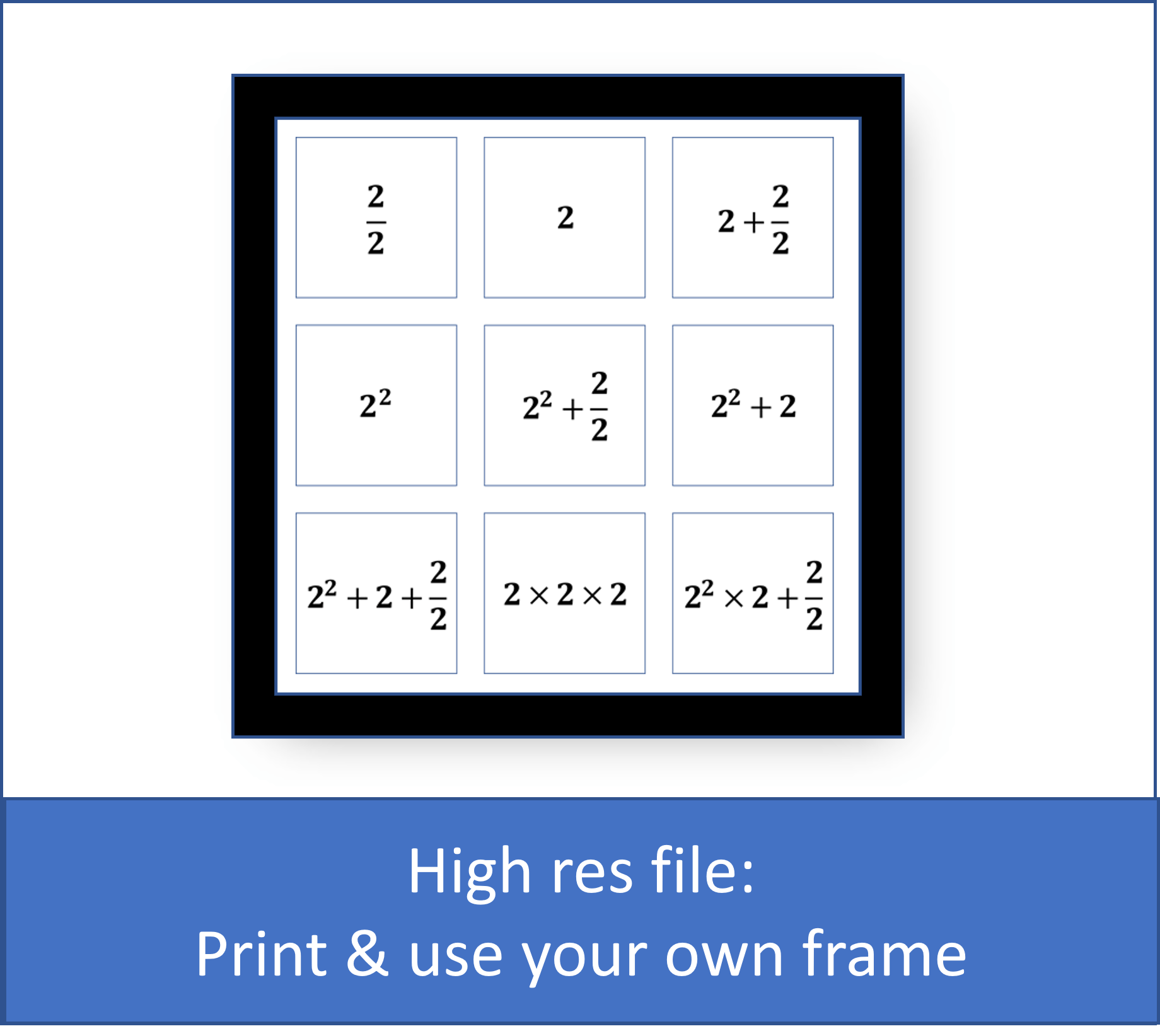# 1 2 3 4 5 6 7 8 9

## We just need a bit of math to help us out.

If you have 2 apples, and need to share them between yourself and a friend - how many apples do you get each?

2 apples shared between 2 friends = 1

Yes - 2/2 = 1## A more difficult one: How to get to 4

Square is when you multiply a number as many times as the square (the little number in a raised position after the main number).

This means 2 multiplied by itself 2 times (2 x 2) = 4.## Combining + and – and x and ÷ and square

Fortunately, there are some simple rules in mathematics helping us to figure out how to solve this one.

The rules are:

1. First you do the squares. So 2x2 = 4
2. Next, you do x and ÷ (mutiply and divsion) , so we do 4 x 2 = 8 AND we do 2/2 = 1
3. As the last thing we do + and –  (plus and minus), so here the 8 from above + 1 (the 2/2) = 9

The rule is Squares before x and ÷ before + and – .## Counting 1 to 9

Here is finally how we can use only 2s to count from 1 to 9:## Related and popular

```The 2-lover’s mug € 22 Add to basketPrint your own Wall Decoration € 10 Add to basketCounting 1-9 wall decoration € 49 – € 69 Select options ```
``` ```